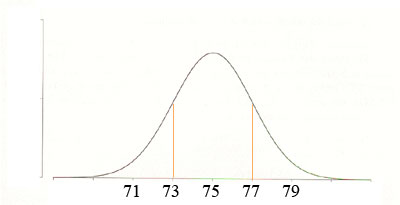SEARCH HOMEMath Central Quandaries & QueriesQuestion from crystal, a student: A population forms a normal distribution with a mean of 75 and a standard deviation of 20. How do I sketch the distribution of sample means for samples of n= 100?Crystal,

If a normal distribution has mean μ and standard deviation σ and you take random samples of size n then the sample means follow a normal distribution with mean μ and standard deviation σ/√n. You have μ = 75, σ = 20 and n = 100 and hence the distribution of sample means is normal with μ = 75 and σ = 20/√100 = 20/10 = 2.HarleyMath Central is supported by the University of Regina and The Pacific Institute for the Mathematical Sciences.